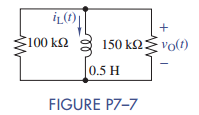### Create an Account

Home / Questions / In Figure P7–7 the initial current through the inductor is iL 0 5 mA a Find iL t and vO ...

# In Figure P7–7 the initial current through the inductor is iL 0 5 mA a Find iL t and vO t for t ≥ 0 b Use MATLAB to plot the waveforms for iL t and vO t c Simulate the problem

In Figure P7–7 the initial current through the inductor is iL(0) = 5 mA.

(a) Find iL(t) and vO(t) for t ≥ 0.

(b) Use MATLAB to plot the waveforms for iL(t) and vO (t).

(c) Simulate the problem using Multisim and compare the results to the plots in part (b).Jun 15 2020 View more View LessSubscribe To Get Solution